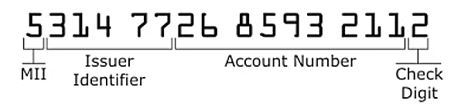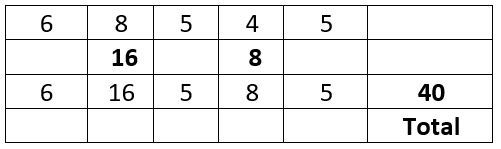# C Programming – HackerRank Solution | Validate Credit Cards |

### (HackerRank) Write a Modular C Programming code to solve Validate Credit Cards, Credit cards use a system of blocked numbers similar to the ISBN

Credit cards use a system of blocked numbers similar to the ISBN. One obvious difference is that the maximum length for the number is 19 digits, although many numbers range from 10-16 digits. For 16-digit card number, the first digit is the Major Industry Identifier (MII) and identifies which group issued the card. The next block of numbers is the issue identifier. Including the MII, the Issue Identifier is 6 digits long. The account number begins with the 7th digit and ends with the next-to-last digit. The final digit is the check digit.Suppose, consider the task of validating the check digit for a credit card with the number 68545 is:To be a valid card number, every even position digit of the card number from right to left is doubled. And Every odd position digit is kept unchanged. Then the sum of all the doubled and unchanged digits is computed. This sum must be evenly divisible by 10. The check digit is 5, the total is 40 and 40 mod 10 is 0. Therefore, this card number is valid. Suppose the check digit is 7, the sum would be 42 which is not divisible by 10. Therefore, card number would be invalid.

TASK: Apply Problem Solving Framework and Write the modular C program to check the validity of a card number.

Input Format

N indicating the Card Number.

Constraints

Consider Card Number as single integer with at least having 2 digits.

Output Format

Display the result whether Card Number is valid / invalid.

Sample Input 0

```68545
```

Sample Output 0

```The card number 68545 is valid.
```

Sample Input 1

```7
```

Sample Output 1

`Card number should have at least 2 digits.`

CODE:

```#include<stdio.h>
#include<stdlib.h>
#include<string.h>
#include<math.h>

int main() {
int card;
int sum=0;
scanf("%d",&card);
int temp=card;
int dig;
int i=1;
if(card<10)
{
printf("Card number should have at least 2 digits.");
}
else{
do
{
dig=card%10;
card=card/10;
if(i%2==0)
{
sum=sum+(2*dig);
}
else{
sum=sum+dig;
}
i++;
}while(card/10!=0);
if(i%2==0)
{
sum+=card*2;
}
else{
sum+=card;
}
if(sum%10==0)
{
printf("The card number %d is valid.",temp);
}
else{
printf("The card number %d is invalid.",temp);
}
}
return 0;
}

```

OUTPUT

```Congratulations, you passed the sample test case.

Click the Submit Code button to run your code against all the test cases.

Input (stdin)

68545

The card number 68545 is valid.
Expected Output

The card number 68545 is valid.```

Please find some more codes of Loops, Condition Statements, 1D Arrays, 2D Arrays, Strings, Pointers, Data Structures, Files, Linked lists, MISC, Solved model question papers & Hacker Rank all solutions on the below page:

Top 100+ C Programming codes – KLE Technological University